Maths-
General
Easy

Question

# What is the factored form of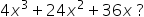Hint:

## The correct answer is: 4x (x + 3) (x + 3) is the factorized form of the given expression.

### Ans:- 4x(x+3)(x+3) is the factorized form of the given expression.Given,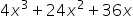Taking out common factor 4 we get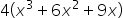Taking out common element x we get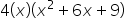Splitting out 6x into 3x+3x we get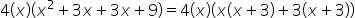Taking out x+3 common out we get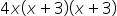As we get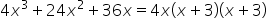∴ 4x (x + 3)(x + 3) is the factorized form of the given expression.#### With Turito Foundation.#### Get an Expert Advice From Turito.